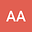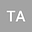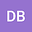Fractional Parabolic Equations with Generalized Mittag-Leffler Kernels
•••• +2
• Abedel-karrem Alomari,
• D. Baleanu,
• Qasem Al-Mdallal
Abedel-karrem Alomari
Yarmouk University

Corresponding Author:abdomari2008@yahoo.com

Author ProfilePrince Sultan University
Author ProfileD. Baleanu
Cankaya University
Author ProfileIn this paper we apply the fractional integrals with arbitrary order depending on the fractional operators of Riemann type (ABR) and Caputo type (ABC) with kernels of Mittag Lefller in three parameters $E_{\alpha,\mu}^\gamma(\lambda,t)$ for solving the time fractional parabolic nonlinear equation. We utilize these operators with homotopy analysis method (HAM) for constructing the new scheme for generating the successive approximations. This procedure are used successfully on two examples for finding the solutions. The effectiveness and accuracy are verified by clarifying the convergence region in the $h$-curves as well as by calculating the residual error and the results were accurate. Depending on this results, this treatment can be used to find the approximate solutions to many fractional differential equations.